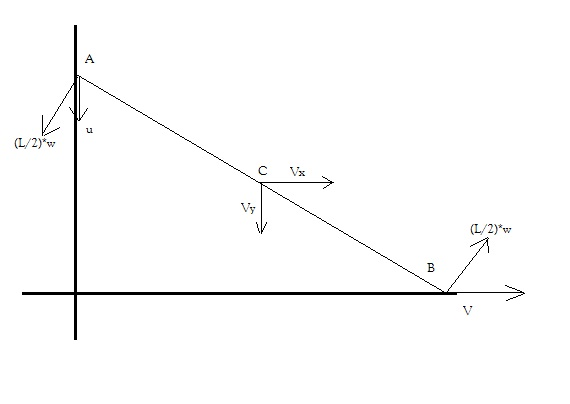#### Thank you for registering.

One of our academic counsellors will contact you within 1 working day.

Click to Chat

1800-5470-145

+91 7353221155

CART 0

• 0
MY CART (5)

Use Coupon: CART20 and get 20% off on all online Study Material

ITEM
DETAILS
MRP
DISCOUNT
FINAL PRICE
Total Price: Rs.

There are no items in this cart.
Continue Shopping

# 1)a solid sphere rolling on a rough horizontal surface with linear speed v collides elastically with a fixed vertical wall.Find the speed of the sphere after it has started pure rolling in backward direction.2)a thin rod of length l leans against a vertical wall and ground.The end B(end resting on ground) is made to move with constant velocity v.Assuming that end A doesnot leave contact with the wall,find the acceleration of geometric centre of the rod when it makes angle thita with the horizontal.plz explain the reason.9 years ago

let pure rolling speed be u

J=m(v+u), where J is linear impulse due to friction

Jr=(2mr2/5)((v+u)/r)

solve for J and u

9 years ago

Hi Ashmita,Refer to the digram above.

Let l be the length of the rod.

Vx,Vy be the horizontal, and vertical components of velocity at any given instant in time of the Centre of mass of the rod.

We will solve this problem with respect to the centre of mass frame of the rod.

For any person on the centre of the rod, the rod will exhibit pure circular motion at any given point in time.

At this instant let us say, the angular velocity of the rod wrt centre of the rod is w.

Let x (=thita) be the angle made by the rod with the horizontal at the end B.

Now for end B:

v - Vx = (l/2)w*sinx

and Vy = (l/2)w*cosx

And for end A:

u - Vy = (l/2)w*cosx

and Vx = (l/2)w*sinx-----------(substitute this in the first equation)

Solving, we get Vx = v//2 & Vy = (v/2)cotx, w = (v/l)*cosecx

So now, the velocity of centre of the rod, at any instant (in vector notation) is:

V = v/2(i) - (v/2)cotx(j)

So acn of the centre of mass is a = dv/dt = [-(v/2)(-cosec^2 x)dx/dt]j

Where dx/dt = (-w) = -(v/l)cosecx.

Hence a = [(-v2/2l)cosec3x]j

Hence a = (v2/2l)cosec3x in the downward direction.

Hope this  helps. Also hope there is no calculation mistake.

Regards,

9 years ago

Hi Ashmita,

Hope you can view the diagram now. Have attached it in a different format.Hope this helps.

x is the angle made at the end B with the horizontal.

Regards,

9 years ago

Hi Ashnita,

Assuming that the vertical wall with which the sphere collides is smooth. Else this cannot be solved without knowing the co-efficient of friction.

Here note, during collision there is only a linear impulse between the vertical wall and the sphere. This Force that causes the impulse passes through the centre of the sphere. Hence there is no angular impulse in this problem.

So angular velocity would remain the same, just after and before collision.

Say w was the angular velocity of sphere before collision (which would be clockwise).

As there was pure rolling before collision w = v/R --------(R is the radius of sphere).

Now after collision (where the collision is elastic), the velocity of the centre of mass of the sphere will become v in the opposite direction, and w would remain the same in the clockwise direction.

And hence the point of contact will start sliping now. And after some time t, pure rolling will start.

The problem is now this (after collision)

The velocity of the centre of mass is v, and w(clockwise) is w=v/R.

Find the pure rolling speed of the sphere.

Write Newtons laws and solve. At the time when pure rolling starts the velocity will be 3v/7.

Hope this helps.

Best Regards,